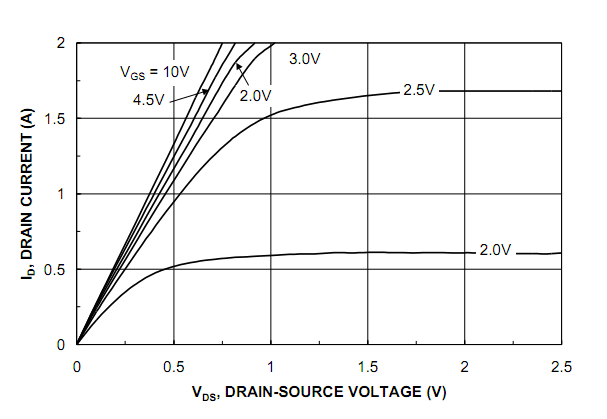# Interpreting MOSFET ID/Vds curve for a MOSFET Switch

In summary, when choosing a logic-level MOSFET as a switch for an IC working at 3.3V, it is important to ensure that the MOSFET can provide a low enough voltage at the drain for the IC to read as low. This can be determined by looking at the drain current vs. V(DS) graph in the datasheet of the MOSFET and using the Rds(on) value at a gate voltage of 2.5V and a drain current of 500mA to calculate the maximum possible Vds. This value can then be adjusted for temperature using Figure 3 in the datasheet.

Hello,

I'm considering using a logic-level MOSFET as a switch in a design. The drain of the MOSFET will be connected to an IC. The IC works at 3.3V and the maximum voltage at which it's guaranteed to read a low is just 0.8V.

Now, I think every MOSFET will able to satisfy this but I'm just trying to understand how can I tell? From looking at the datasheet of http://www.fairchildsemi.com/ds/FD%2FFDG6317NZ.pdf" [Broken], the drain current vs. V(DS) graph seems relevant.Let's assume I need a current of 500mA through the MOSFET. If I look at the above graph, it suggests that when Id = 500mA, the drain to source voltage should be around about 0.25V, for a gate voltage of 3V. Is my analysis correct?

Last edited by a moderator: